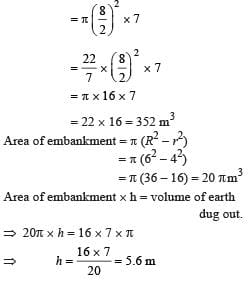Test Description

## 10 Questions MCQ Test Mathematical Olympiad Class 8 | Olympiad Test: Achieves Section-2

Olympiad Test: Achieves Section-2 for Class 8 2023 is part of Mathematical Olympiad Class 8 preparation. The Olympiad Test: Achieves Section-2 questions and answers have been prepared according to the Class 8 exam syllabus.The Olympiad Test: Achieves Section-2 MCQs are made for Class 8 2023 Exam. Find important definitions, questions, notes, meanings, examples, exercises, MCQs and online tests for Olympiad Test: Achieves Section-2 below.
 1 Crore+ students have signed up on EduRev. Have you?
Olympiad Test: Achieves Section-2 - Question 1

### What is the value of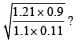Detailed Solution for Olympiad Test: Achieves Section-2 - Question 1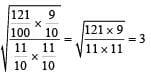Olympiad Test: Achieves Section-2 - Question 2

### 9x2 + 25 - 30x is the square of

Detailed Solution for Olympiad Test: Achieves Section-2 - Question 2

9x2 + 25 - 30x
= (3x)2 + (5)2 - 2 (3x) (5)
= (3x - 5)2

Olympiad Test: Achieves Section-2 - Question 3

### One of the factor of x2 + 17x + 60 is

Detailed Solution for Olympiad Test: Achieves Section-2 - Question 3

x2 + 17x + 60
=x2 +5x +12x + 60
=x(x+5) +12(x+5)
=(x+5) + (x+12)

Olympiad Test: Achieves Section-2 - Question 4

The perimeter of a rectangle is 54 cm. If its width is 2 cm more than one-fourth of its length. What is its length?

Detailed Solution for Olympiad Test: Achieves Section-2 - Question 4

Let the length be x.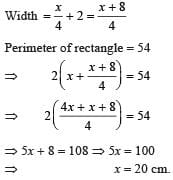Olympiad Test: Achieves Section-2 - Question 5

One of the letters from the word SOCIOLOGY is chosen at random. What is the probability that this letter is O?

Detailed Solution for Olympiad Test: Achieves Section-2 - Question 5

Total number of letters = 9
Number of letter ‘O’ = 3
Required probability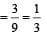Olympiad Test: Achieves Section-2 - Question 6

What is the value of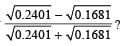Detailed Solution for Olympiad Test: Achieves Section-2 - Question 6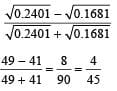Olympiad Test: Achieves Section-2 - Question 7

The product of two numbers is 1575 and their quotient is 9/7. What is the difference of the numbers?

Detailed Solution for Olympiad Test: Achieves Section-2 - Question 7

Let the numbers be x and y.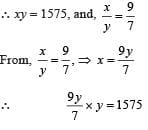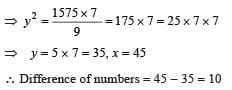Olympiad Test: Achieves Section-2 - Question 8

The diagonal of a square is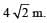What is its perimeter?

Detailed Solution for Olympiad Test: Achieves Section-2 - Question 8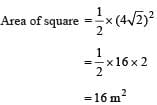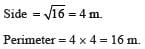Olympiad Test: Achieves Section-2 - Question 9

What is the simplified value of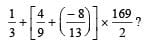Detailed Solution for Olympiad Test: Achieves Section-2 - Question 9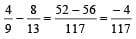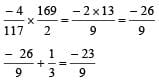Olympiad Test: Achieves Section-2 - Question 10

A well with internal diameter 8m is dug 7m deep. The earth taken out of it is spread at around to a width of 2 m to form an embankment. The height of embankment will be :

Detailed Solution for Olympiad Test: Achieves Section-2 - Question 10

Volume of earth dug out